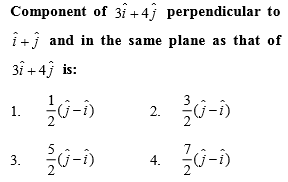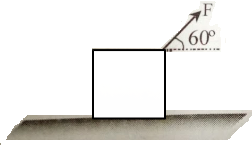Complete Question Bank + Test Series
Complete Question Bank

Difficulty Level:

A child pulls a box with a force of  at an angle of $60°$above the horizontal. Then the horizontal and vertical components of the force are -(A)  100 N, 175 N

(B)  86.6 N, 100 N

(C)  100 N, 86.6 N

(D)  100 N, 0 N

Difficulty Level:

A man rows a boat with a speed of  in northwest direction. The shoreline makes an angle of $15°$ south of west. Obtain the component of the velocity of the boat along the shoreline.

(A)

(B)

(C)

(D)

Complete Question Bank + Test Series
Complete Question Bank

Difficulty Level:

If  . Find component of $\stackrel{\to }{\mathrm{A}}$ along $\stackrel{\to }{\mathrm{B}}$ & perpendicular to $\stackrel{\to }{\mathrm{B}}$.

(A)

(B)

(C)

(D)